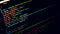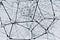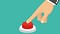# How to create tensorflow tfrecords out of any dataset:

## A complete step by step tutorial for absolute beginners with exampleImage by dlanor_s: Unsplash

When working with deep learning models, we often use very large datasets. It can be very useful to store them in a binary file format that takes up less space and so improve training time. The TFRecord format is a simple format in tensorflow for storing a sequence of binary records. I am going to take through the process of creating a tfrecord, step by step. But before we dive into that, we need to understand something called protocol buffers

# So, what is a protocol buffer?

A protocol buffer (or protobuf) is for efficient serialization of structured data. Imagine you have two services written in two…

# A Gentle Introduction to Backpropagation and Implementing Neural Network AnimationSource: Photo by Alina Grubnyak, on Unsplash

At its core, a neural network is an algorithm that was designed to learn patterns in real-life data and make predictions. An important part of this learning is done using the backpropagation algorithm. The backpropagation attempts to correct errors at each layer to make a better prediction. We can do this by fine-tuning the weights of the neural network. This fine-tuning mechanism is done by the partnership of gradient descent and backpropagation algorithms.

The point of backpropagation is based on the intuition that we can improve the final choice (output) by correcting every small decision that leads to it.

Once…

# Activation functions: Why are they important and how to use them?Source

# Activation Functions:

When you build a neural network, one of the decisions you can make is the choice of an activation function. Activation functions give neural networks the power of mapping nonlinear functions. Imparting non-linearity to the neural network helps it to solve complex problems. They help your model to capture squiggly looking patterns that you will often encounter in real-life data. This is essential since nature in general doesn’t always operate linearly.

Simply put, the activation function squishes the output of the summation operator into a different range of values that represent how much a node should contribute.

# What should we look for in an activation function?

• Don’t vanish: A…

# Understanding Gradient Descent and breaking down the math behind:

Gradient descent is a widely used optimization algorithm used by a range of machine learning algorithms. Machine learning algorithms have cost functions that compute the error (predicted value — actual value) of the model. So, the best model has to be the one that has the minimum cost. The goal of the gradient descent is to find parameters of the model that has the minimum cost. When we pass the model that we would like to use to Gradient descent, it will compute the cost with different parameters for the model and return the parameters for which the cost is…

# Understanding Adaboost and Scikit-learn’s algorithm:

Ensemble models and more particularly Boosting algorithms have become quite popular on online data science competition forums like Kaggle. Ensemble learning is the method of using a group of models to make our prediction. Today we are going to talk about an ensemble boosting algorithm called AdaBoost. If you aren’t familiar with what ensemble means, you can refer to my previous article on ensemble models where I will give a brief introduction to the idea of ensembles and 4 main techniques that ensemble models use. …

# Ensemble Learning Methods in Machine Learning

Ensemble learning is a compelling technique that helps machine learning systems improve their performance. The technique gained a lot of popularity in the online data science competition platform Kaggle with a good number of winning solutions using ensembling methods. It uses a group of models to predict rather than just using one model. It’s kind of like how you look at reviews before you buy something. You read the reviews, weigh how many people like the product vs. how many people don’t, and decide ultimately whether the product is worth your money, right? That’s exactly what ensemble learning does. …

# Logistic Regression: Understand the math behind the algorithm

Logistic regression is a supervised binary classification algorithm. You’re probably wondering why its called logistic regression then, it's only because it uses the concept of regression to classify. If that doesn’t make sense to you yet, don't worry, we’re going to break it all down. In logistic regression, the target value that we want to predict has the value of zero or one. It either belongs to a class, so the value is 1 or it doesn’t, in which case the value is 0 and the data distribution is binomial. The goal of the algorithm is to model the probability…

# Types of Distance Metrics and Using User Defined Distance metrics in Scikit’s KNN Algorithm:

KNN algorithm is one of the most commonly used and important algorithms in data science. It is a supervised classification algorithm, meaning that the data we feed into the algorithm is labeled and we use them to classify the data based on their similarities. The algorithm works by first measuring the distance of each point to k nearest points and assigns the label of the closest point. It is used for classifying images, species, etc.

The most important part of a KNN algorithm is the distance metric it uses. Thankfully scikit allows us to tweak this part. …